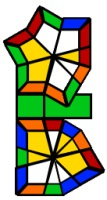# Square-1 Scrambler

The Square-1 is a shape changing twisty puzzle which is also used on official WCA competitions. This scrambler generates a random permutation for this puzzle and displays an image how it will look after you have applied these rotations to an unscrambled Square-1.

Use the following settings:Scrambles: Set how many scrambles you want to generate.

Length: Set the length of one scramble. In the case of the Square-1 if this number is odd then the middle layer is twisted and it is not square shaped as seen from above. To see how the unscrambled puzzle looks, set this number to 0.

Separate: Activating this checkbox the program will insert separators after every five slice into the algorithm for a better readability.

The Orient and FRU only settings are inactive for the Square-1. The color scheme is the following: up white, bottom yellow, left orange, front green, right red, back blue.

## The Notation of the Square-1

The upper and bottom layers of the Square-1 can be rotated in 30° steps. A 180° right side slice can be done if the position of the top and bottom layer pieces allow this.

When you generate a scramble for the Square-1 you can see pairs of numbers followed by a slash. The first number refers to the top and the second number refers to the bottom layer and each show how many steps you have to turn on the certain face. One step is the thickness of a thin piece (an edepiece of the unscrambled cube). Notice that the thick piece (a cornerpiece) is exactly twice as big as the thin piece. So there are always 12 steps on the upper and 12 steps on the bottom face forming a full circle, so one step is exactly 30°. A positive number means a clockwise turn and a negative means a counterclockwise turn.
Some examples:

• 1, 0 / - Turn the upper layer one step (30°) clockwise and do a slice.
• 0, -2 / - Turn the bottom layer two steps (60°) counterclockwise and do a slice.
• -2, 3 / - Turn the top layer counterclockwise two step, the bottom layer clockwise three step and then do a middle slice.